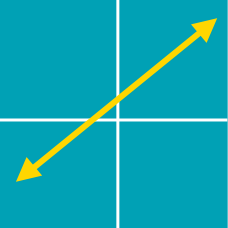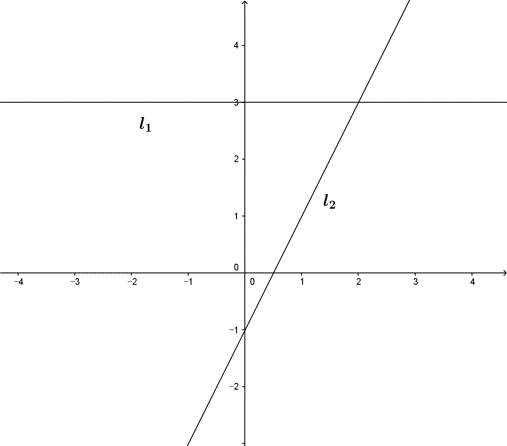Algebra

# Linear Equations - Intersection of LinesIn the graph above, $l_1$ is a horizontal line with $y$-intercept $3$, and $l_2$ has a slope of $2$ with $y$-intercept $-1$. Find the coordinates of the intersection point of the two lines.

What is the interval of all possible values of $a$ such that the two lines $x+y=\lvert a \rvert$ and $ax-y=196$ intersect in the first quadrant?

Consider the line passing through two points $(5, 0)$ and $(0, -5)$. Consider another line passing through two points $(-4, 0)$ and $(0, -2)$. If these two lines and a third line $y=ax-85$ intersect at one point, what is the value of the constant $a$?

A line passing through the intersection point of the two lines $x+2y+3=0\;\;\text{and}\;\; 2x-y+\frac{a}{2}=0$ also passes through two other points $(9,\ -8)\$ and $\ (-17,\ 9)$. What is the value of $a$?

Suppose the intersection point of the two lines $x-2y-9=0, 2x+3y-53=0$

is $P=(a,b)$. What is the value of $a+b$?

×